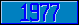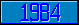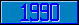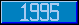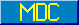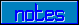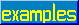## \$QSUBSCRIPT

### 7.1.5.14 \$QSubscript

This form returns a value which is derived from namevalue. If namevalue has the form NAME( s1 , s2 , ... , sn ), considering n to be zero if there are no subscripts, and m is the value of intexpr, then \$QSubscript(namevalue, intexpr) is defined as follows:

1. Values of m less than –1 are reserved for possible future use by extension of the standard.
2. If m = –1, the result is the environment if namevalue includes an environment; otherwise the empty string.
3. If m = 0, the result is NAME without an environment even if one is present.
4. If m > n, the result is the empty string.
5. Otherwise, the result is the subscript value denoted by sm.

Note that the namevalue is not "executed", and will not affect the naked indicator, nor generate an error if the namevalue represents an undefined glvn. The arguments are evaluated in left to right order, and the naked indicator will only be affected by the last gvn reference (if any) executed while evaluating them.